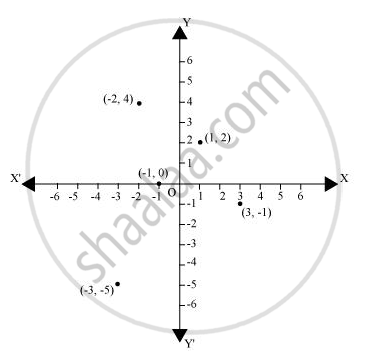# n which quadrant or on which axis do each of the points (– 2, 4), (3, – 1), (– 1, 0), (1, 2) and (– 3, – 5) lie? Verify your answer by locating them on the Cartesian plane. - Mathematics

In which quadrant or on which axis do each of the points (– 2, 4), (3, – 1), (– 1, 0), (1, 2) and (– 3, – 5) lie? Verify your answer by locating them on the Cartesian plane.

#### SolutionThe point (-2,4) lies in the IInd quadrant in the Cartesian plane because for point(-2, 4), x-coordinate is negative and y-coordinate is positive.

Again, the point (3, -1) lies in the IVth quadrant in the Cartesian plane because for point(3,-1), x-coordinate is positive and y-coordinate is negative.

The point(-1,0) lies on negative x-axis because for point (-1,0), the value of y-coordinate is zero and the value of x-coordinate is negative.

The point (1,2) lies in the Ist quadrant as for point (1, 2), both x and y are positive.

The point (-3, -5) lies in the IIIrd quadrant in the Cartesian plane because for point (-3, -5), both xand y are negative.

Concept: Plotting a Point in the Plane If Its Coordinates Are Given.
Is there an error in this question or solution?
Chapter 3: Coordinate Geometry - Exercise 3.3 [Page 65]

#### APPEARS IN

NCERT Class 9 Maths
Chapter 3 Coordinate Geometry
Exercise 3.3 | Q 1 | Page 65

Share